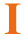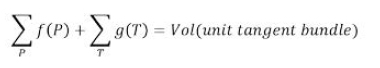THE SINGAPORE MAGAZINE OF RESEARCH, TECHNOLOGY AND EDUCATION About Innovation
 COVER STORY: Identities on Hyperbolic Surfaces A Discussion of Some Identities on Hyperbolic Surfaces and their Applications by Tan Ser Peowt has long been known that closed (no boundary), orientable (two-sided) surfaces are classified topologically by their genus. For example, the genus zero surface is a sphere, the genus one surface is a torus (doughnut with one hole), the genus two surface is a doughnut with two holes, etc. (see Figure 1). It turns out that one can endow these surfaces with constant curvature metrics. For example, the sphere has a spherical metric (curvature +1) much like the surface of a smooth beach ball, and the torus has flat Euclidean metrics (curvature 0), which are flat in the usual Euclidean sense. These are well known to players of computer games like Pacman where opposite sides of a Euclidean rectangle (the playing area) are identified. In fact, such a game is taking place over a flat torus. We may also identify opposite sides of a parallelogram instead; this is still topologically a torus but with a different flat Euclidean metric on it (see Figure 2).It turns out that surfaces of genus 2 are the most interesting. In these cases, one can put metrics of constant curvature -1 on these surfaces (called hyperbolic metrics), that is, every point on the surface looks like a saddle point. Furthermore, there is a whole parameter space of such metrics that can be put on a surface of genus g. To be precise, the space of hyperbolic metrics is 6g-6 dimensional. For surfaces with boundary, one can also put hyperbolic metrics on most of these surfaces in such a way that the boundaries are geodesic (the technical term for a straight line in a metric space is a geodesic). The space of all hyperbolic structures on a surface (with or without boundary) is called the moduli space of the surface and this has a very rich geometric structure in itself. It is the subject of intense investigation by many mathematicians as it lies in the confluence of several different branches of mathematics like hyperbolic geometry, algebraic geometry, complex analysis, dynamical systems, mathematical physics and number theory. In some sense, these are some of the most basic geometric objects in mathematics, much like the elementary particles in Physics. Over the last couple of decades, several interesting and beautiful identities concerning the lengths of orthogeodesics/closed geodesics on hyperbolic surfaces with boundary have been discovered by various authors. Remarkably, these identities hold even when one varies the hyperbolic structure on the surface. Furthermore, some of these identities have found surprising applications in such diverse areas like low dimensional geometry and topology, algebraic geometry and mathematical physics. In particular, we highlight the remarkable work of Maryam Mirzakhani  where she generalized the McShane identity  and used it to compute the Weil-Petersson volumes of the moduli spaces of bordered Riemann surfaces, and also to give a new proof of the Kontsevich-Witten Theorem about the intersection numbers of stable classes on the moduli space, first conjectured by Witten and proven by Kontsevich. (Mirzakhani recently became the first female recipient of the Fields Medal, widely regarded as the mathematical version of the Nobel prize; both Kontsevich and Witten were previous recipients of the Fields Medal). Besides the McShane identity , and various generalizations, there have been related identities due to Basmajian  and Bridgeman . All of these identities have as a starting point the fact that the surface has some boundary components (i.e. not closed surfaces). For several years, a basic question was whether or not one could produce identities for general closed hyperbolic surfaces. Perhaps a good analogy for this is the process of creation of pearls by oysters. Often, it is much easier to create a pearl by introducing some impurity into the oyster, from which the pearl is built around; it is often difficult for the oyster to spontaneously create a pearl without this initial impurity. The boundary of the surface plays the part of the impurity in this analysis. The identities are often built around these boundaries and if one starts with a surface without boundary, it is not clear where to start to build an identity around. Recently, the author together with his collaborator Feng Luo from Rutgers, were able to do this. The results have been published in the Journal of Differential Geometry . A more detailed and technical survey of these identities and the way they are related can be found in the survey article by Bridgeman and the author . Hyperbolic surfaces differ markedly from flat Euclidean surfaces. One example of such a difference is seen in the celebrated result due to Joan Birman and Caroline Series, whereby the set of simple (no self-intersection) closed geodesics (coming back to itself with the same direction) on a hyperbolic surface is very sparse on the surface. That is, in a very strong sense, most points on the surface do not lie on one of these geodesics. Technically, they proved that this set has Hausdorff dimension zero. Another very important result for hyperbolic surfaces is the ergodicity of the geodesic flow, which roughly speaking, says that if one takes a generic point on the surface and a generic direction and generate a geodesic path, then one can get arbitrarily close to every point on the surface with any direction. Both these results fail spectacularly in the case of flat Euclidean surfaces. The unifying idea behind the proofs of all these identities (Basmajian, Bridgeman, McShane, and Luo-Tan) is fairly simple. One considers a set X with a finite measure coming from the hyperbolic structure. For example, if the surface is a hyperbolic surface with one boundary component of length L, then we may consider X to be the boundary, whose measure is given by the length L. Alternatively, we may let X be the unit tangent bundle of the surface, which basically means the set of all pairs (x,v), where x is a point on the surface, and v is a direction. Again, one may put a natural measure on this set. This would be the total area of the surface (coming from the choice of the point x) multiplied by 2 (coming from the choice of the direction v). We then try to decompose the set X in a geometric way into a countable disjoint union of subsets Xi. Usually, there is some leftover which we denote by the set Z. In general, the set Z is extremely complicated, but by using the hyperbolicity of the surface (the Birman-Series result or the ergodicity of the geodesic flow alluded to earlier), we can somehow show that Z has measure 0. The countable sets are in general indexed by some geometric objects, for example, the orthogeodesics on the surface, the simple closed curves, or embedded simple sub-surfaces. The identity then follows by taking the sum of the measures of Xi which is now the measure of X. Technically, calculating the measures of the Xi can be very challenging. The Basmajian identity comes from decomposing the boundary of the surface. It takes the formwhere the sum is over all othogeodesics and g is a function on ||, the lengths of , which is easily determined. The Bridgeman identity comes from decomposing the unit tangent bundle of the surface. It takes the formwhere the sum is over the same index set as the Basmajian identity, the function h is a dilogarithm function and computed explicitly by Bridgeman, and the right hand side is now the volume of the unit tangent bundle. The McShane identity comes from decomposing X, a connected component of the boundary of the surface, the decomposition being different from that of the Basmajian identity since it is obtained by different geometric considerations. It takes the formwhere the sum is over all embedded three-holed spheres P which contain X as a boundary, L is the length of X and the function G is a function of the lengths of the three boundary geodesics of P and computed explicitly in  and . The Luo-Tan identity by Feng Luo and the author is obtained by decomposing the unit tangent bundle of the surface to subsets which are indexed by either embedded three-holed spheres P or one-holed tori T in the surface. In some sense, the key insight here is that since there are no distinguished boundary components to work with, and no distinguished starting point, we choose all possible points and all possible directions as our starting point. The key technical step to obtain the result is to compute the measures of these subsets. The computation turns out to be technically much more challenging than for the previous identities but is still possible. One then obtains an identity of the formwhere the right hand side is the volume of the unit tangent bundle of the surface, and on the left hand side, the first sum is over all embedded geometric three-holed spheres P, and the second sum is over all embedded geometric one-holed tori T in the surface. Both of these sums are infinite sums in general (since there are infinitely many embedded Ps and Ts in general), and the functions f and g are dilogarithm functions defined on the lengths of the simple closed geodesics on the simple surfaces P and T and determined explicitly in . As we mentioned at the beginning, these identities hold throughout the moduli space of hyperbolic structures on the surfaces, and hence say something about the geometry of the moduli spaces. The challenge is to use them to shed new light on the geometry of these moduli spaces, much in the way that Mirzakhani did in . Another challenge of course is to discover the many new and interesting identities on hyperbolic surfaces and manifolds waiting to be revealed. Tan Ser Peow is a Professor in the Department of Mathematics at the National University of Singapore. For more information about this article, please contact Professor Tan at mattansp@nus.edu.sg